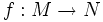# Local isometry of complete Riemannian manifolds is covering map

Suppose$M$ and$N$ are complete Riemannian manifolds, and$f:M \to N$ is a local isometry. Then,$f$ is a covering map. In particular, if$N$ is simply connected, then$f$ is an isometry.